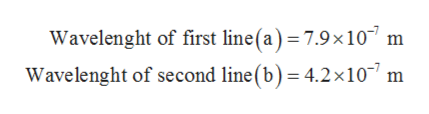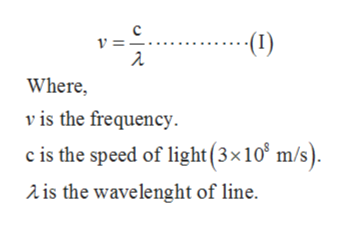# When rubidium ions are heated to a high temperature, two lines are observed in its line spectrum at wavelengths (a) 7.9 × 10−7 m and (b) 4.2 × 10−7 m. What are the frequencies of the two lines? What color do we see when we heat a rubidium compound?

Question
199 views

When rubidium ions are heated to a high temperature, two lines are observed in its line spectrum at wavelengths (a) 7.9 × 10−7 m and (b) 4.2 × 10−7 m. What are the frequencies of the two lines? What color do we see when we heat a rubidium compound?

check_circle

Step 1

Frequencies and wavelengths are related to each other via the equation shown below.

Step 2

Given data:help_outlineImage TranscriptioncloseWavelenght of first line (a) 7.9x10 m Wavelenght of second line (b)= 4.2x107 m fullscreen
Step 3

The Frequencies of two lines are calcul...help_outlineImage Transcriptionclosec (1) Where vis the frequency. c is the speed of light (3x10 m/s). is the wavelenght of line. fullscreen

### Want to see the full answer?

See Solution

#### Want to see this answer and more?

Solutions are written by subject experts who are available 24/7. Questions are typically answered within 1 hour.*

See Solution
*Response times may vary by subject and question.
Tagged in

### Chemistry